# Bypass Capacitor: What is it's importance in an amplifier circuit?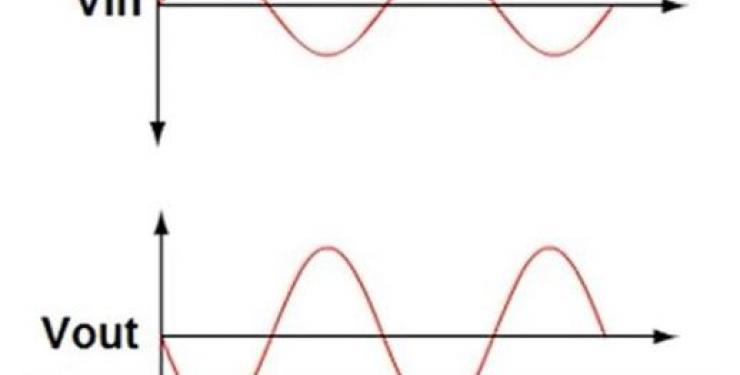In this blog, we will review the bypass capacitor, its function, and how it is important in an amplifier circuit.

## What is a Bypass Capacitor?

A bypass capacitor is added to an amplifier circuit in order to allow AC signals to bypass the emitter resistor. This effectively removes it from the output gain equation resulting in an increase to the amplifiers AC gain.

## What is a common-emitter amplifier circuit?

The basic voltage divider bias common-emitter amplifier circuit consists of four resistors, and an NPN bipolar junction transistor. In addition to these core components, there are two coupling capacitors which effectively block the DC current from input signal as well as the output signal. The signal that appears at the Vin of the circuit is amplified, and phase shifted by 180 degrees when it reaches Vout.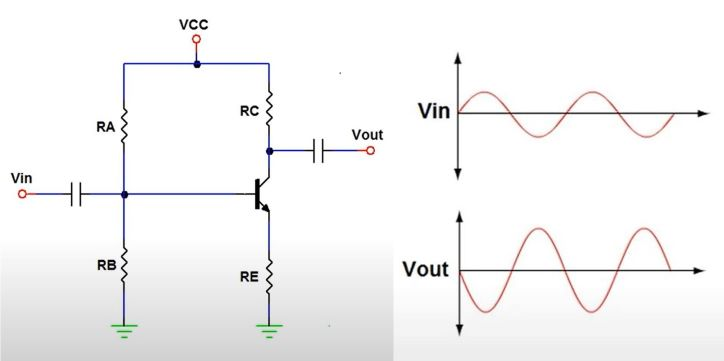We will go through an example with assigned values to better evaluate how great the AC voltage gain would be if a bypass capacitor was added to the circuit.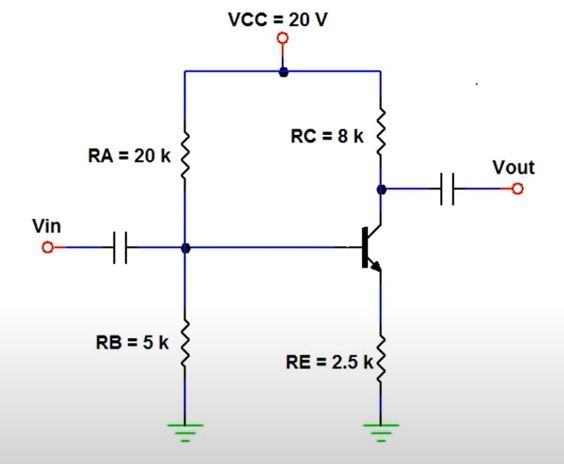## Bypass capacitor effects on a common-emitter amplifier circuit:

Step 1: To begin, we must calculate the base voltage, VB, of the circuit without the bypass capacitor. We must apply the voltage divider rule using the values RA, RB, and VCC.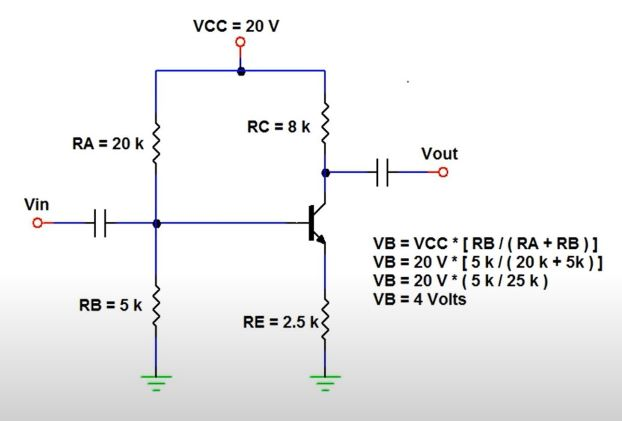Step 2: Using the VB value obtained, we can then calculate the VE at the transistor. This is done by subtracting the base emitter voltage, VBE, from the calculated VB. A typical value of VBE is 0.7 V.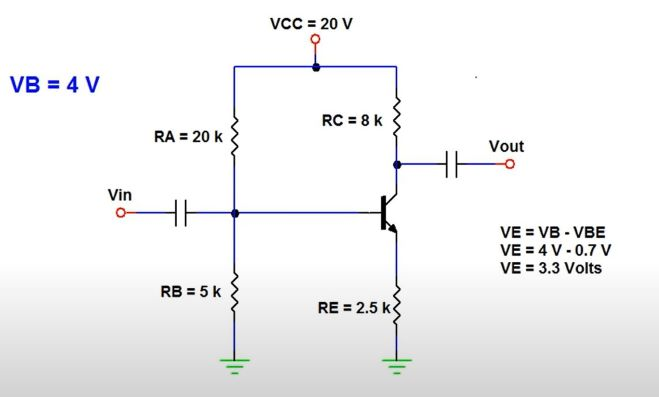Step 3: The next step is to use Ohm’s Law to calculate the emitter current, IE using resistor RE, and VE.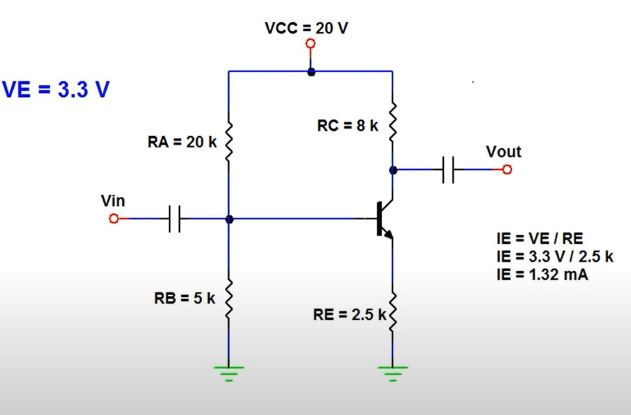Step 4: Now we must calculate the internal emitter resistance, re, of the BJT. This value will vary with current and is approximated using a 25 mV voltage drop, the calculated IE, and using Ohm’s law.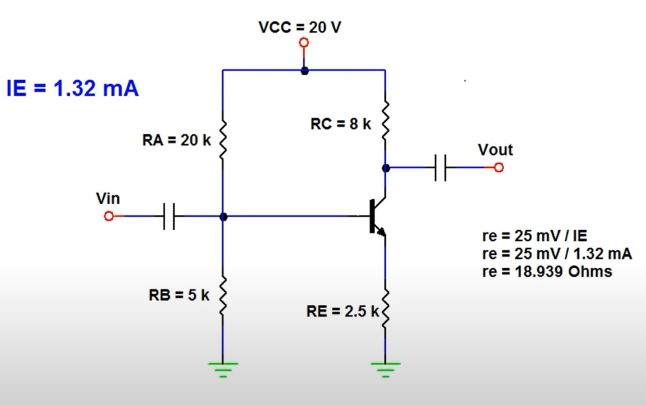Step 5: Calculate the voltage gain for the amplifier with, and without the bypass capacitor. Using a simplified relationship equation, we can calculate voltage gain without a bypass capacitor using the values we calculated.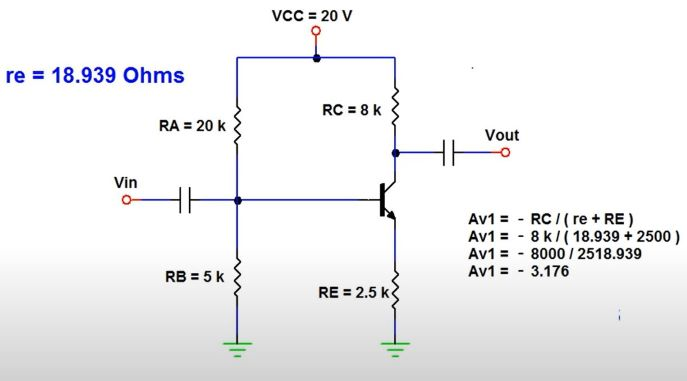For a circuit with a bypass capacitor, we will disregard resistor RE, as it is now bypassed for AC signals and does not contribute to the voltage gain. Using another simplified equation, we can calculate the voltage gain for the modified circuit.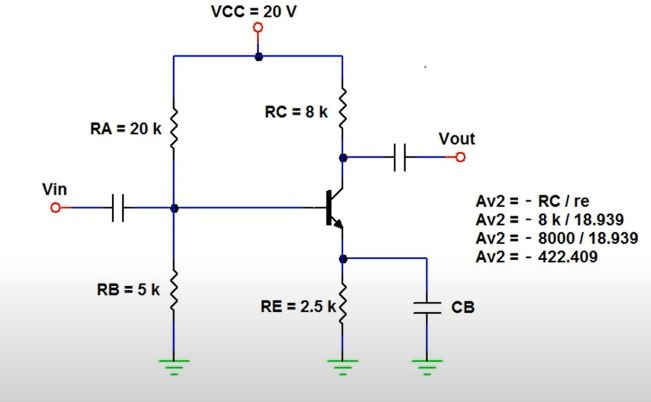Step 6: We can now compare the voltage gains calculated with or without the bypass capacitor by using a ratio of the two values.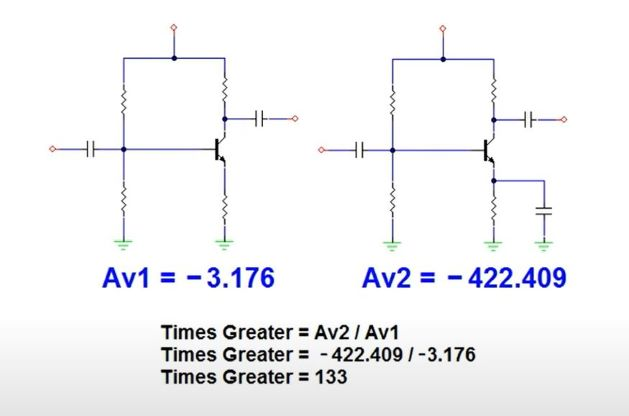In conclusion, we can see that a bypass capacitor causes a significantly higher AC voltage gain in amplifier circuit 2, thus pulling more AC voltage than amplifier circuit 1.

We hope that this has been helpful as a student or practicing Electronics technician. If you have any questions regarding the Electronics Technician program, feel free to get in touch with us at info@gbctechtraining.com or give us a call at 1-888-553-5333 to speak with a Program Consultant.